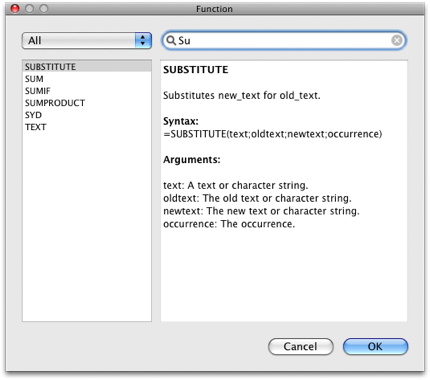Calculations and Functions

Tables offers you a wide range of calculation options and many functions from different areas.

Starting from simple operations like addition, subtraction, division, multiplication and involution until numerical compares like less, greater, equal or unequal up to more than 120 functions from the areas:

Mathematics, Trigonometry, Financial, Statistic, Matrix, Date & Time, Text, Logical, Spreadsheet and Information